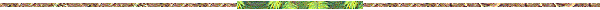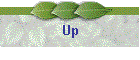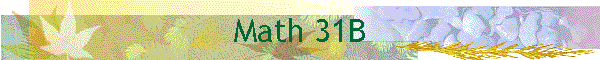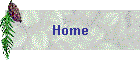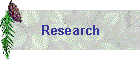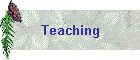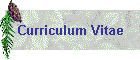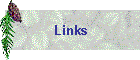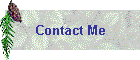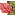Course: Math 31B, Calculus and Analytic GeometryText: J. Stewart, Calculus, 5th Ed., Brooks/Cole Pub. Co.Schedule of Lectures: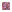Section 6.5: Review of integration, average value of a functionSection 8.7: Numerical integration, trapezoidal rule, Simpson's rule, error estimatesSection 6.1: Areas between curvesSection 6.2: VolumesSection 6.3: Volumes by cylindrical shellsSection 6.4: WorkSection 7.1: Inverse functionsSection 7.2-4: Exponential and logarithmic functionsSection 10.4: Exponential growth and decaySection 7.5-6: Inverse trigonometric functions; hyperbolic functionsSection 7.7: Indeterminate formsSection 8.1: Integration by partsSection 12.12: Taylor's theorem with remainderSection 8.2-3: Trigonometric integrals; trigonometric substitutionSection 8.4: Partial fractionsSection 8.8: Improper integralsSection 9.1-2: Arc length, area of surface of revolutionSection 10.1-2: Differential equations, direction fieldsSection 10.3: Separable DE's, homogeneous DE'sSection 10.6: First-order linear DE's